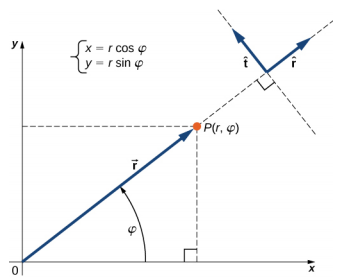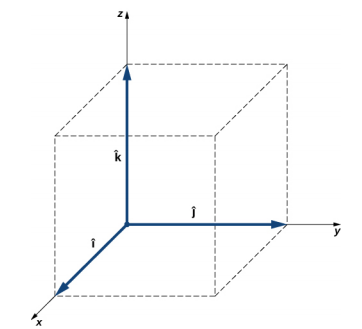$$\require{cancel}$$

# 3.5: Coordinate Systems and Components of a Vector (Part 2)

•• OpenStax
• OpenStax
$$\newcommand{\vecs}{\overset { \rightharpoonup} {\mathbf{#1}} }$$ $$\newcommand{\vecd}{\overset{-\!-\!\rightharpoonup}{\vphantom{a}\smash {#1}}}$$$$\newcommand{\id}{\mathrm{id}}$$ $$\newcommand{\Span}{\mathrm{span}}$$ $$\newcommand{\kernel}{\mathrm{null}\,}$$ $$\newcommand{\range}{\mathrm{range}\,}$$ $$\newcommand{\RealPart}{\mathrm{Re}}$$ $$\newcommand{\ImaginaryPart}{\mathrm{Im}}$$ $$\newcommand{\Argument}{\mathrm{Arg}}$$ $$\newcommand{\norm}{\| #1 \|}$$ $$\newcommand{\inner}{\langle #1, #2 \rangle}$$ $$\newcommand{\Span}{\mathrm{span}}$$ $$\newcommand{\id}{\mathrm{id}}$$ $$\newcommand{\Span}{\mathrm{span}}$$ $$\newcommand{\kernel}{\mathrm{null}\,}$$ $$\newcommand{\range}{\mathrm{range}\,}$$ $$\newcommand{\RealPart}{\mathrm{Re}}$$ $$\newcommand{\ImaginaryPart}{\mathrm{Im}}$$ $$\newcommand{\Argument}{\mathrm{Arg}}$$ $$\newcommand{\norm}{\| #1 \|}$$ $$\newcommand{\inner}{\langle #1, #2 \rangle}$$ $$\newcommand{\Span}{\mathrm{span}}$$

## Polar Coordinates

To describe locations of points or vectors in a plane, we need two orthogonal directions. In the Cartesian coordinate system these directions are given by unit vectors $$\hat{i}$$ and $$\hat{j}$$ along the x-axis and the y-axis, respectively. The Cartesian coordinate system is very convenient to use in describing displacements and velocities of objects and the forces acting on them. However, it becomes cumbersome when we need to describe the rotation of objects. When describing rotation, we usually work in the polar coordinate system.

In the polar coordinate system, the location of point P in a plane is given by two polar coordinates (Figure $$\PageIndex{1}$$). The first polar coordinate is the radial coordinate r, which is the distance of point P from the origin. The second polar coordinate is an angle $$\varphi$$ that the radial vector makes with some chosen direction, usually the positive x-direction. In polar coordinates, angles are measured in radians, or rads. The radial vector is attached at the origin and points away from the origin to point P. This radial direction is described by a unit radial vector $$\hat{r}$$. The second unit vector $$\hat{t}$$ is a vector orthogonal to the radial direction $$\hat{r}$$. The positive + $$\hat{t}$$ direction indicates how the angle $$\varphi$$ changes in the counterclockwise direction. In this way, a point P that has coordinates (x, y) in the rectangular system can be described equivalently in the polar coordinate system by the two polar coordinates (r, $$\varphi$$). Equation 2.4.13 is valid for any vector, so we can use it to express the x- and y-coordinates of vector $$\vec{r}$$. In this way, we obtain the connection between the polar coordinates and rectangular coordinates of point P:

$\begin{cases} x = r \cos \varphi \\ y = r \sin \varphi \end{cases} \ldotp \label{2.18}$Figure $$\PageIndex{1}$$: Using polar coordinates, the unit vector $$\hat{r}$$ defines the positive direction along the radius r (radial direction) and, orthogonal to it, the unit vector $$\hat{t}$$ defines the positive direction of rotation by the angle $$\varphi$$.

Example $$\PageIndex{1}$$: Polar Coordinates

A treasure hunter finds one silver coin at a location 20.0 m away from a dry well in the direction 20° north of east and finds one gold coin at a location 10.0 m away from the well in the direction 20° north of west. What are the polar and rectangular coordinates of these findings with respect to the well?

Strategy

The well marks the origin of the coordinate system and east is the +x-direction. We identify radial distances from the locations to the origin, which are rS = 20.0 m (for the silver coin) and rG = 10.0 m (for the gold coin). To find the angular coordinates, we convert 20° to radians: 20° = $$\frac{\pi\; 20}{180}$$ = $$\frac{\pi}{9}$$. We use Equation \ref{2.18} to find the x- and y-coordinates of the coins.

Solution

The angular coordinate of the silver coin is $$\varphi_{S}$$ = $$\frac{\pi}{9}$$, whereas the angular coordinate of the gold coin is $$\varphi_{G}$$ = $$\pi$$ − $$\frac{\pi}{9}$$ = $$\frac{8 \pi}{9}$$. Hence, the polar coordinates of the silver coin are (rS, $$\varphi_{S}$$) = (20.0 m, $$\frac{\pi}{9}$$) and those of the gold coin are (rG, $$\varphi_{G}$$) = (10.0 m, \frac{8 \pi}{9}\)). We substitute these coordinates into Equation \ref{2.18} to obtain rectangular coordinates. For the gold coin, the coordinates are

$\begin{cases} x_{G} = r_{G} \cos \varphi_{G} = (10.0\; m) \cos \frac{8 \pi}{9} = -9.4\; m \\ y_{G} = r_{G} \sin \varphi_{G} = (10.0\; m) \sin \frac{8 \pi}{9} = 3.4\; m \end{cases} \Rightarrow (x_{G}, y_{G}) = (-9.4\; m, 3.4\; m) \ldotp$

For the silver coin, the coordinates are

$\begin{cases} x_{S} = r_{S} \cos \varphi_{S} = (20.0\; m) \cos \frac{\pi}{9} = 18.9\; m \\ y_{S} = r_{S} \sin \varphi_{S} = (20.0\; m) \sin \frac{\pi}{9} = 6.8\; m \end{cases} \Rightarrow (x_{S}, y_{S}) = (18.9\; m, 6.8\; m) \ldotp$

## Vectors in Three Dimensions

To specify the location of a point in space, we need three coordinates (x, y, z), where coordinates x and y specify locations in a plane, and coordinate z gives a vertical positions above or below the plane. Three-dimensional space has three orthogonal directions, so we need not two but three unit vectors to define a three-dimensional coordinate system. In the Cartesian coordinate system, the first two unit vectors are the unit vector of the x-axis $$\hat{i}$$ and the unit vector of the y-axis $$\hat{j}$$. The third unit vector $$\hat{k}$$ is the direction of the z-axis (Figure $$\PageIndex{2}$$). The order in which the axes are labeled, which is the order in which the three unit vectors appear, is important because it defines the orientation of the coordinate system. The order x-y-z, which is equivalent to the order $$\hat{i}$$ - $$\hat{j}$$ - $$\hat{k}$$, defines the standard right-handed coordinate system (positive orientation).Figure $$\PageIndex{2}$$: Three unit vectors define a Cartesian system in three-dimensional space. The order in which these unit vectors appear defines the orientation of the coordinate system. The order shown here defines the right-handed orientation.

In three-dimensional space, vector $$\vec{A}$$ has three vector components: the x-component $$\vec{A}_{x}$$ = Ax $$\hat{i}$$, which is the part of vector $$\vec{A}$$ along the x-axis; the y-component $$\vec{A}_{y}$$ = Ay $$\hat{j}$$, which is the part of $$\vec{A}$$ along the y-axis; and the z-component $$\vec{A}_{z}$$ = Az $$\hat{k}$$, which is the part of the vector along the z-axis. A vector in three-dimensional space is the vector sum of its three vector components (Figure $$\PageIndex{3}$$):

$\vec{A} = A_{x} \hat{i} + A_{y} \hat{j} + A_{z} \hat{k} \ldotp \label{2.19}$

If we know the coordinates of its origin b(xb, yb, zb) and of its end e(xe ye, ze), its scalar components are obtained by taking their differences: Ax and Ay are given by

$\begin{cases} A_{x} = x_{e} - x_{b} \nonumber \\ A_{y} = y_{e} - y_{b} \ldotp \nonumber \end{cases}$

and the z-component is given by

$A_{z} = z_{e} - z_{b} \ldotp \label{2.20}$

Magnitude A is obtained by generalizing Equation 2.4.8 to three dimensions:

$A = \sqrt{A_{x}^{2} + A_{y}^{2} + A_{z}^{2}} \ldotp \label{2.21}$

This expression for the vector magnitude comes from applying the Pythagorean theorem twice. As seen in Figure $$\PageIndex{3}$$, the diagonal in the xy-plane has length $$\sqrt{A_{x}^{2} + A_{y}^{2}}$$ and its square adds to the square Az2 to give A2. Note that when the z-component is zero, the vector lies entirely in the xy-plane and its description is reduced to two dimensions.Figure $$\PageIndex{3}$$: A vector in three-dimensional space is the vector sum of its three vector components.

Example $$\PageIndex{2}$$: Takeoff of a Drone

During a takeoff of IAI Heron (Figure $$\PageIndex{4}$$), its position with respect to a control tower is 100 m above the ground, 300 m to the east, and 200 m to the north. One minute later, its position is 250 m above the ground, 1200 m to the east, and 2100 m to the north. What is the drone’s displacement vector with respect to the control tower? What is the magnitude of its displacement vector?Figure $$\PageIndex{4}$$: The drone IAI Heron in flight. (credit: SSgt Reynaldo Ramon, USAF)

Strategy

We take the origin of the Cartesian coordinate system as the control tower. The direction of the +x-axis is given by unit vector $$\hat{i}$$ to the east, the direction of the +y-axis is given by unit vector $$\hat{j}$$ to the north, and the direction of the +z-axis is given by unit vector $$\hat{k}$$, which points up from the ground. The drone’s first position is the origin (or, equivalently, the beginning) of the displacement vector and its second position is the end of the displacement vector.

Solution

We identify b(300.0 m, 200.0 m, 100.0 m) and e(480.0 m, 370.0 m, 250.0m), and use Equation 2.4.4 and Equation \ref{2.20} to find the scalar components of the drone’s displacement vector:

$\begin{cases} D_{x} = x_{e} - x_{b} = 1200.0\; m - 300.0\; m = 900.0\; m,\\ D_{y} =y_{e} - y_{b} = 2100.0\; m - 200.0\; m = 1900.0\; m, \\ D_{z} =z_{e} - z_{b} = 250.0\; m - 100.0\; m = 150\; m \ldotp \end{cases}$

We substitute these components into Equation \ref{2.19} to find the displacement vector:

$\vec{D} = D_{x}\; \hat{i} + D_{y}\; \hat{j} + D_{z}\; \hat{k} = 900.0\; \hat{i} + 1900.0\; \hat{j} + 150.0\; \hat{k} = (0.90\; \hat{i} + 1.90\; \hat{j} + 0.15\; \hat{k})\; km \ldotp$

We substitute into Equation \ref{2.21} to find the magnitude of the displacement:

$D = \sqrt{D_{x}^{2} + D_{y}^{2} + D_{z}^{2}} = \sqrt{(0.90\; km)^{2} + (1.90\; km)^{2} + (0.15\; km)^{2}} = 4.44\; km \ldotp$

Exercise 2.7

If the average velocity vector of the drone in the displacement in Example 2.7 is $$\vec{u}$$ = (15.0 $$\hat{i}$$ + 31.7 $$\hat{j}$$ + 2.5 $$\hat{k}$$) m/s, what is the magnitude of the drone’s velocity vector?

3.5: Coordinate Systems and Components of a Vector (Part 2) is shared under a CC BY license and was authored, remixed, and/or curated by OpenStax.